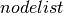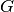Warning

This documents an unmaintained version of NetworkX. Please upgrade to a maintained version and see the current NetworkX documentation.

# to_scipy_sparse_matrix¶

to_scipy_sparse_matrix(G, nodelist=None, dtype=None, weight='weight', format='csr')[source]

Return the graph adjacency matrix as a SciPy sparse matrix.

Parameters: G (graph) – The NetworkX graph used to construct the NumPy matrix. nodelist (list, optional) – The rows and columns are ordered according to the nodes in. Ifis None, then the ordering is produced by G.nodes(). dtype (NumPy data-type, optional) – A valid NumPy dtype used to initialize the array. If None, then the NumPy default is used. weight (string or None optional (default=’weight’)) – The edge attribute that holds the numerical value used for the edge weight. If None then all edge weights are 1. format (str in {‘bsr’, ‘csr’, ‘csc’, ‘coo’, ‘lil’, ‘dia’, ‘dok’}) – The type of the matrix to be returned (default ‘csr’). For some algorithms different implementations of sparse matrices can perform better. See  for details. M – Graph adjacency matrix. SciPy sparse matrix

Notes

The matrix entries are populated using the edge attribute held in parameter weight. When an edge does not have that attribute, the value of the entry is 1.

For multiple edges the matrix values are the sums of the edge weights.

Whendoes not contain every node in, the matrix is built from the subgraph ofthat is induced by the nodes in.

Uses coo_matrix format. To convert to other formats specify the format= keyword.

The convention used for self-loop edges in graphs is to assign the diagonal matrix entry value to the weight attribute of the edge (or the number 1 if the edge has no weight attribute). If the alternate convention of doubling the edge weight is desired the resulting Scipy sparse matrix can be modified as follows:

>>> import scipy as sp
>>> G = nx.Graph([(1,1)])
>>> A = nx.to_scipy_sparse_matrix(G)
>>> print(A.todense())
[]
>>> A.setdiag(A.diagonal()*2)
>>> print(A.todense())
[]


Examples

>>> G = nx.MultiDiGraph()